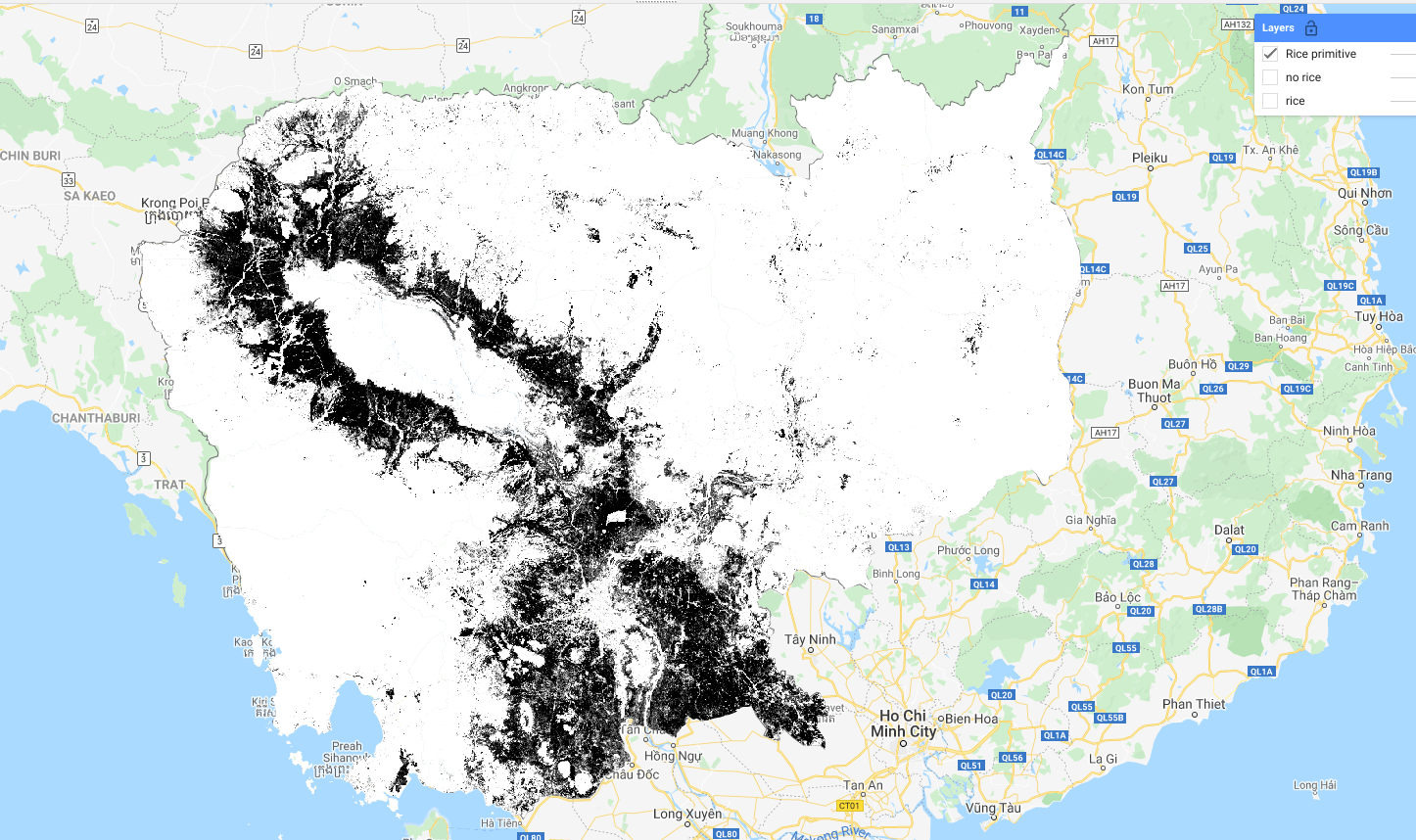# Rice Mapping using machine learning in Code Editor

Building a Random Forest model

Step 1: open the code editor by going to https://code.earthengine.google.com/

Step 2: Import Data from Google Earth Engine Asset with the code below

```// import the composite
var composites = ee.ImageCollection("projects/servir-mekong/yearlyComposites");
// import the training data
var data = ee.FeatureCollection("projects/servir-mekong/referenceData/riceTraining");

```

Step 3: Filter rice and non_rice data from training dataset for visualization and add the layers to the Map

```// Select rice and no rice
var rice = data.filter(ee.Filter.eq('land_class',1))
var non_rice = data.filter(ee.Filter.eq('land_class',0))

//Show Rice and Non_Rice Data Layer
```

Step 4:  Sample the training data (only one year) with regional composite to train in the machine learning algorithm.

```var y = 2018;
var start = ee.Date.fromYMD(y,1,1);
var end = ee.Date.fromYMD(y,12,31);

var image = ee.Image(composites.filterDate(start,end).first()).divide(10000);

var trainingData  = data.filter(ee.Filter.eq("year",y));
print("Training Sample",trainingData.size())
var trainingSample = image.sampleRegions({collection:trainingData,properties:["land_class"],scale:30,geometries:true});
```

Step 5:  Train the training sample in random forest for primitive and Show the classification results in Map

```var bandNames = image.bandNames();
print(bandNames)
var classifier = ee.Classifier.randomForest(25,0).setOutputMode('PROBABILITY').train(trainingSample,"land_class",bandNames);

var classification = image.classify(classifier).multiply(100)

1.Qianqian Han says:
2.thisearthsite says: